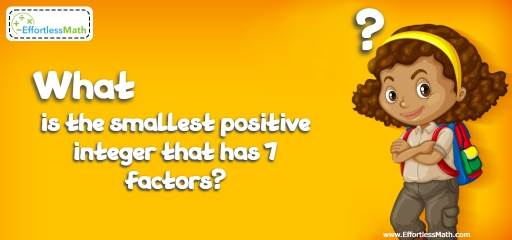# Other Topics Puzzle – Challenge 97

This is a great mathematics puzzle and brain teaser which contains some mathematical content. Can you solve it? The full solution is also given.## Challenge:

What is the smallest positive integer that has 7 factors?

### The Absolute Best Book to Challenge Your Smart Student!

To find the answer, we can factorize numbers from 1, one by one. But, it takes for ever!
Every integer N is the product of powers of prime numbers:
N $$= P^{a}Q^{b}…R^{y}$$
Where P, Q, …,R are prime numbers and a, b, …, y are positive integers.
If N is a power of a prime, then N $$= p^{α}$$, therefore, it has α + 1 factors.
If N $$= P^{a}Q^{b}…R^{y}$$, then, N has (a+1) (b+1) … (y+1) factors.
To find the smallest number that has 7 factors, first write the factors of seven: 7 = 1 × 7
It means that the number in this question has just one prime factor in its decomposition – one with the exponent of α = 6. Keep in mind that b = 0, and $$Q^{b} = Q^{0} = 1$$
N $$= P^{6}Q^{0}$$. To make N as small as possible, we have to choose the smallest available prime 2. The answer is obviously $$N = 2^{6} = 64$$.
The seven factors of 64 are: 1, 2, 4, 8, 16, 32 and 64

The Best Books to Ace Algebra

### What people say about "Other Topics Puzzle – Challenge 97 - Effortless Math: We Help Students Learn to LOVE Mathematics"?

No one replied yet.

X
45% OFF

Limited time only!

Save Over 45%

SAVE $40 It was$89.99 now it is \$49.99